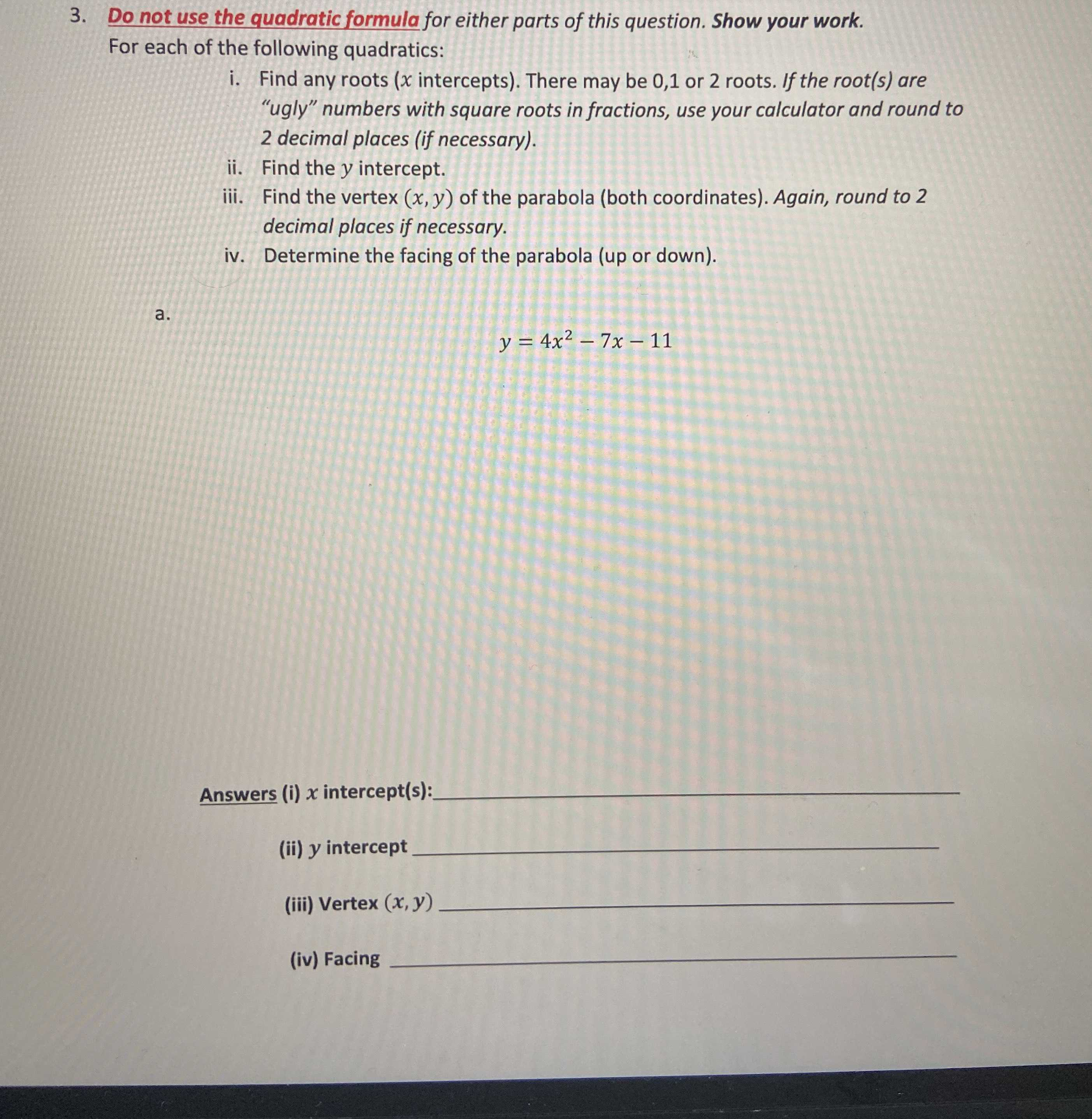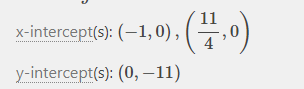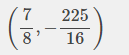### Still have math questions?

Arithmetic
Question3. Do not use the quadratic formula for either parts of this question. Show your work. For each of the following quadratics:

i. Find any roots $$( x$$ intercepts). There may be $$0,1$$ or $$2$$ roots. If the root(s) are "ugly" numbers with square roots in fractions, use your calculator and round to$$2$$ decimal places (if necessary).

ii. Find the $$y$$ intercept.

iii. Find the vertex $$( x , y )$$ of the parabola (both coordinates). Again, round to $$2$$ decimal places if necessary.

iv. Determine the facing of the parabola (up or down).

$$y = 4 x ^ { 2 } - 7 x - 11$$